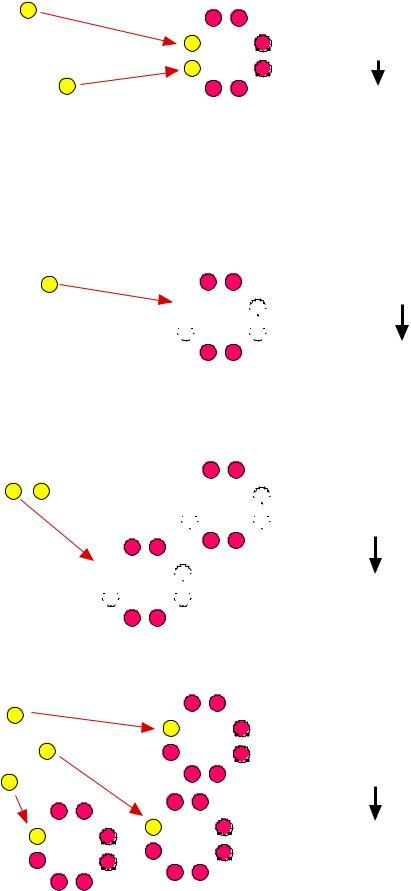## Ionic Bonds Worksheet Answers PDF Details

Ionic Bonds Worksheet Answers can be found in a variety of formats. The most common type of ionic bonds worksheet answer is a PDF. However, you may also find ionic bonds worksheet answers in Excel or Word format. When looking for the correct answer to a bond question, it is important to first determine the type of bond that is being discussed. There are three types of ionic bonds: electrovalent, covalent, and coordinate covalent. Each type has its own unique properties which can be tested using various methods. The best way totest the strength of an ionic bond is usually through melting point or boiling point measurements.

Here is the information about the form you were in search of to fill out. It can show you how much time it will take to complete ionic bonds worksheet answers, exactly what parts you need to fill in, and so on.

Form NameIonic Bonds Worksheet Answers
Form Length4 pages
Fillable?No
Fillable fields0
Avg. time to fill out1 min
Other namescharting oxidation number worksheet, charting oxidation number chapter 7 answers, ionic bonding worksheet answers, charting oxidation number answers

## Form Preview Example

 Bonding Basics - Ionic Bonds Name _______________________ Complete the chart for each element. Element # of Protons # of Electrons # of Valence Electrons Oxidation Number Sodium Chlorine Beryllium Fluorine Lithium Oxygen Phosphorus

Follow your teacher’s directions to complete each ionic bond.

(1) Potassium + Fluorine

(2) Magnesium + Iodine

T. TRIMPE 2002

(3) Sodium + Oxygen

(4) Sodium + Chlorine

(5) Calcium + Chlorine

(6) Aluminum + Chlorine

T. TRIMPE 2002Bonding Basics - Ionic Bonds Answer Key/Teacher Notes

Complete the chart for each element.

 Element # of Protons # of Electrons # of Valence Electrons Oxidation Number Sodium 11 11 -> 10 1 1+ Chlorine 17 17 -> 18 7 1- Beryllium 4 4 -> 2 2 2+ Fluorine 9 9 -> 10 7 1- Lithium 3 3 -> 2 1 1+ Oxygen 8 8 -> 10 6 2- Phosphorus 15 15 -> 18 5 3-

NOTE: I have the students use a red pen/pencil to change the # of electrons to the amount it would be if the valence electrons were removed or added. They can see the difference between the number of protons (+) and electrons (-), which relates to the charge or oxidation number. If the ion has more protons (+), it would be a positive ion. If it has more electrons (-), it would be a negative ion.

Follow your teacher’s directions to complete each ionic bond.

 Step 2 (1) Potassium + Fluorine Step 3 1- Write the symbols for each element. K F 2 - Use Fruity Pebbles (or other cereal/candy with more than one color) to create the Lewis structure for each. Step 1

3 - Draw an arrow (or more if needed) to show the

transfer of electrons and move the cereal to the new location.

4 - Determine the charge for each ion and write the formula.

5 - Make sure the sum of the oxidation numbers is zero and write the chemical formula.

6 - Have the students use a pencil or crayon to draw the electrons as they remove the pieces of cereal.

K1+F1-

KF

K would have a charge of 1+ since it lost an electron

Fluorine would have a charge of 1- since it gained an electron.

(2) Magnesium + Iodine

 Mg I

I

Mg2+ I 12-

MgI2

Mg would have a charge of 2+ since it lost two electrons.

Each I ion would have a charge of 1- since each gained an electron. A subscript “2” is used to show that two ions were used in the bond.

Students will start with one magnesium and one iodine atom. Since the oxidation numbers must equal zero, they will need to add another iodine atom.

T. TRIMPE 2002(3) Sodium + Oxygen

 Na O Na1+2 O 2- Na Na2O Students will start with one sodium and one

oxygen atom. Since the oxidation numbers must equal zero, they will need to add another sodium atom.

(4) Sodium + Chlorine

Each Na ion would have a charge of 1+ since each lost an electron. A subscript “2” is used to show that two ions were used in the bond.

The O ion would have a charge of 2- since it gained two electrons.

Na Cl

Na1+Cl1-

NaCl

Na would have a charge of 1+ since it lost an electron

Cl would have a charge of

1- since it

gained an electron.

(5) Calcium + Chlorine

CaCl

Cl

Ca 2+Cl 12-

CaCl2

Ca would have a charge of 2+ since it lost two electrons.

Each Cl ion would have a charge of 1- since each gained an electron. A subscript “2” is used to show that two ions were used in the bond.

(6) Aluminum + Chlorine

 Al Cl Cl Cl

Al3+Cl13-

AlCl3

The Al ion would have a charge of 3+

since it lost three electrons.

Each Cl ion would have a charge of 1- since each gained an electron. A subscript “3” is used to show

that three ions were used in the bond.

T. TRIMPE 2002

## Watch Ionic Bonds Worksheet Answers Video Instruction

If you believe this page is infringing on your copyright, please familiarize yourself with and follow our DMCA notice and takedown process - click here to proceed .